Ex 9.3

Chapter 9 Class 11 Sequences and Series (Term 1)
Serial order wise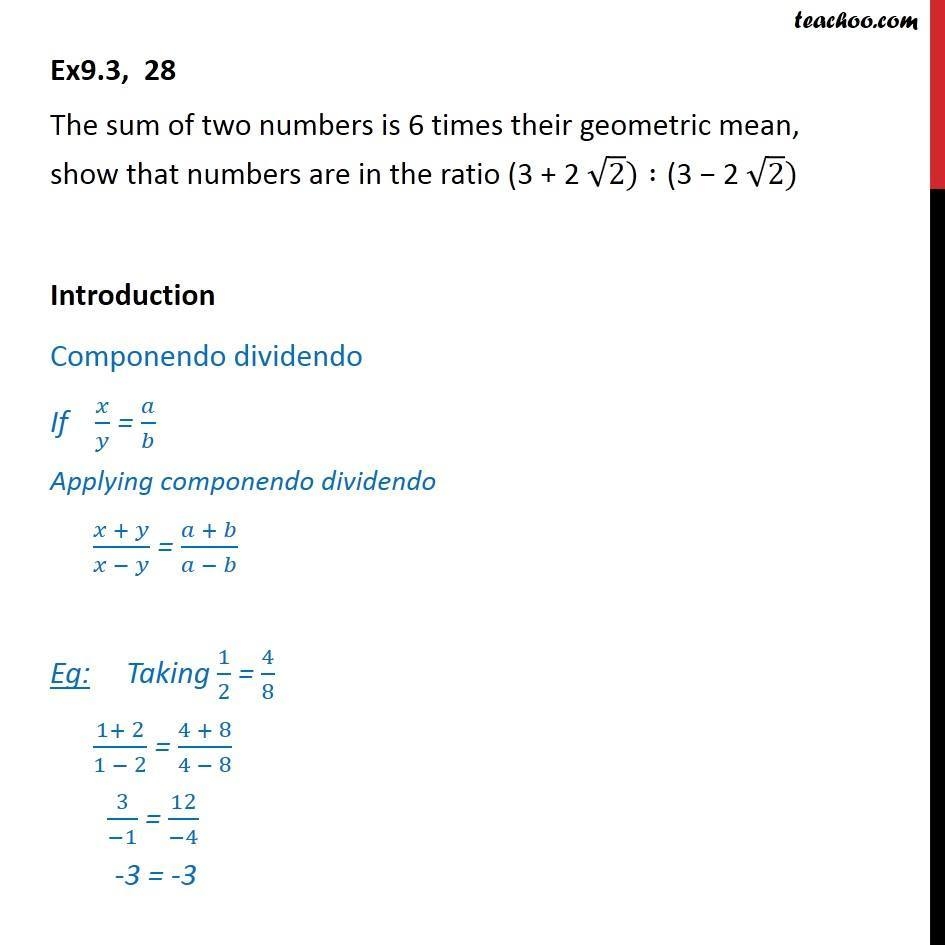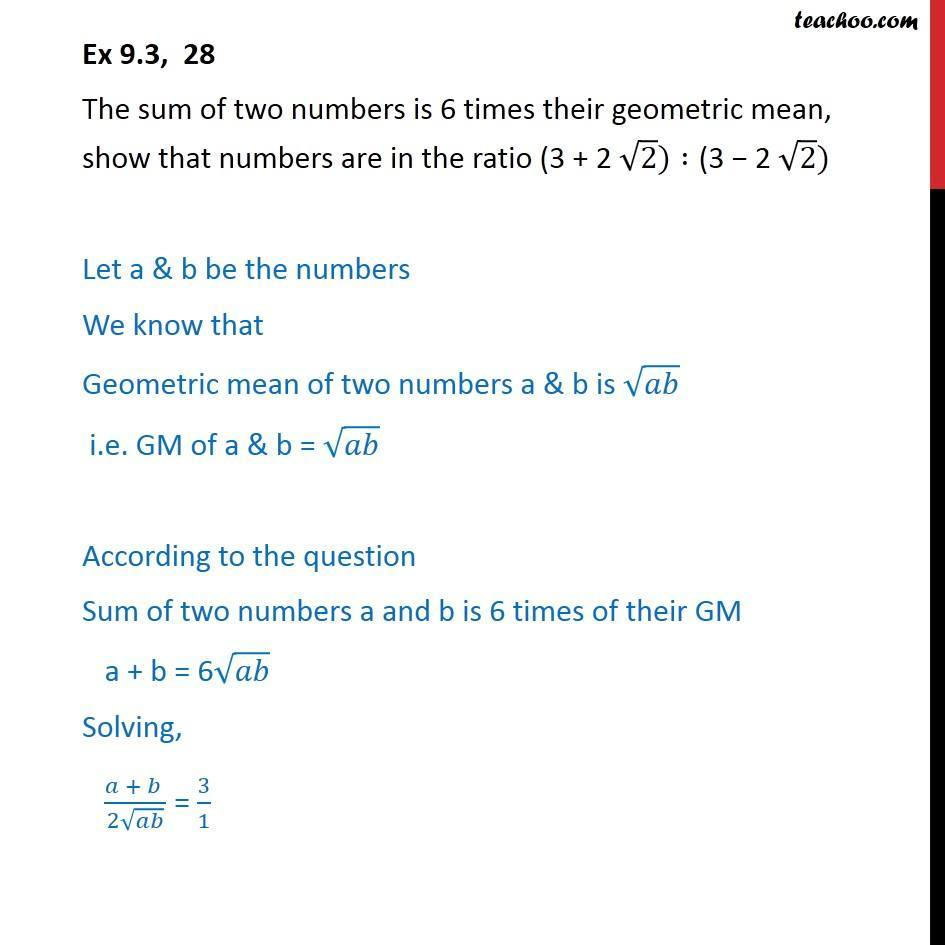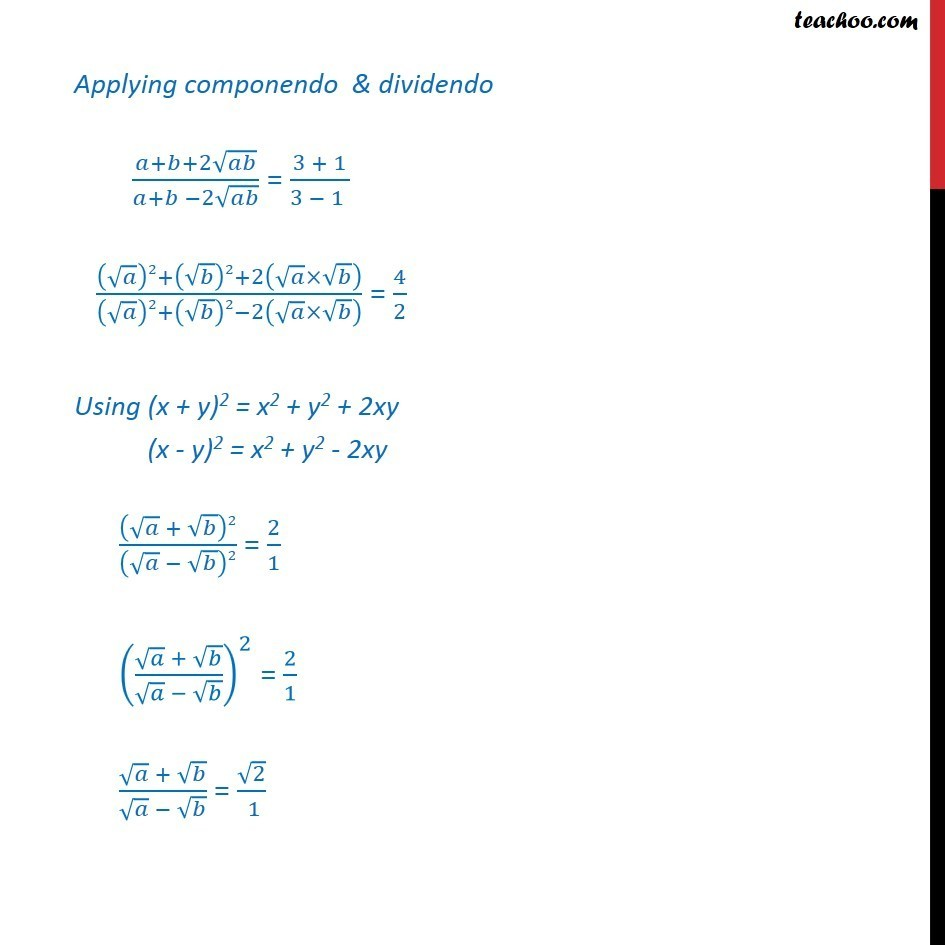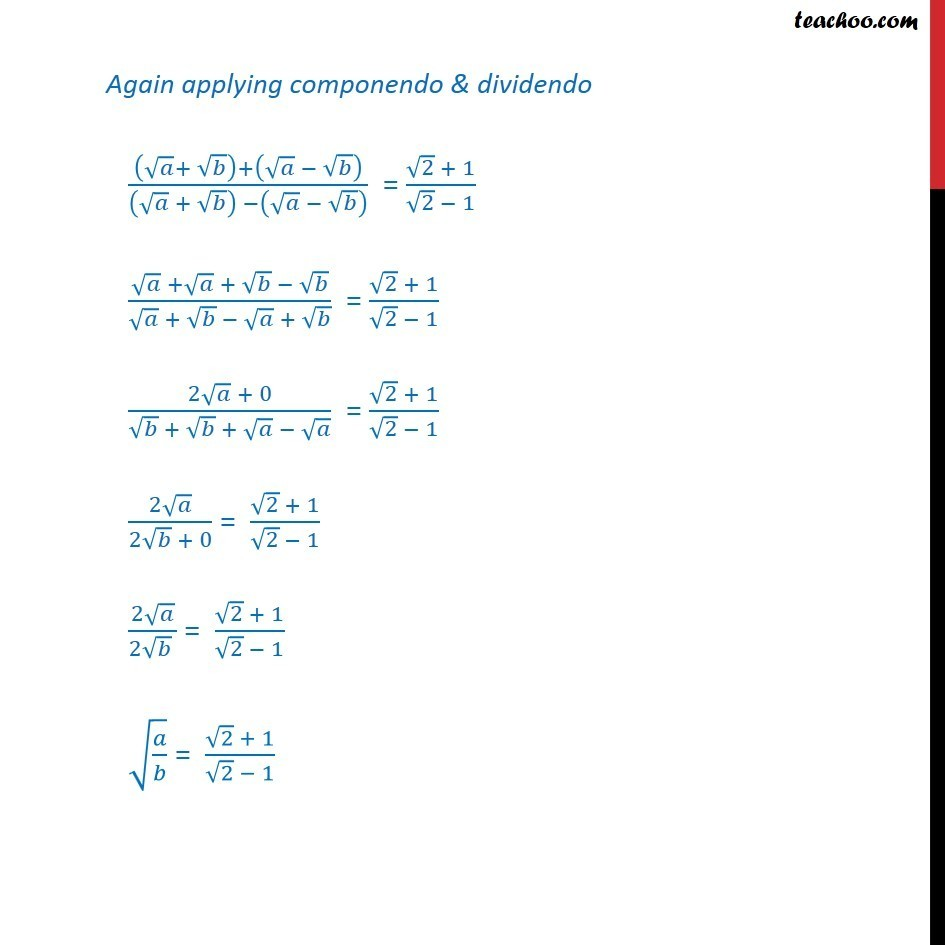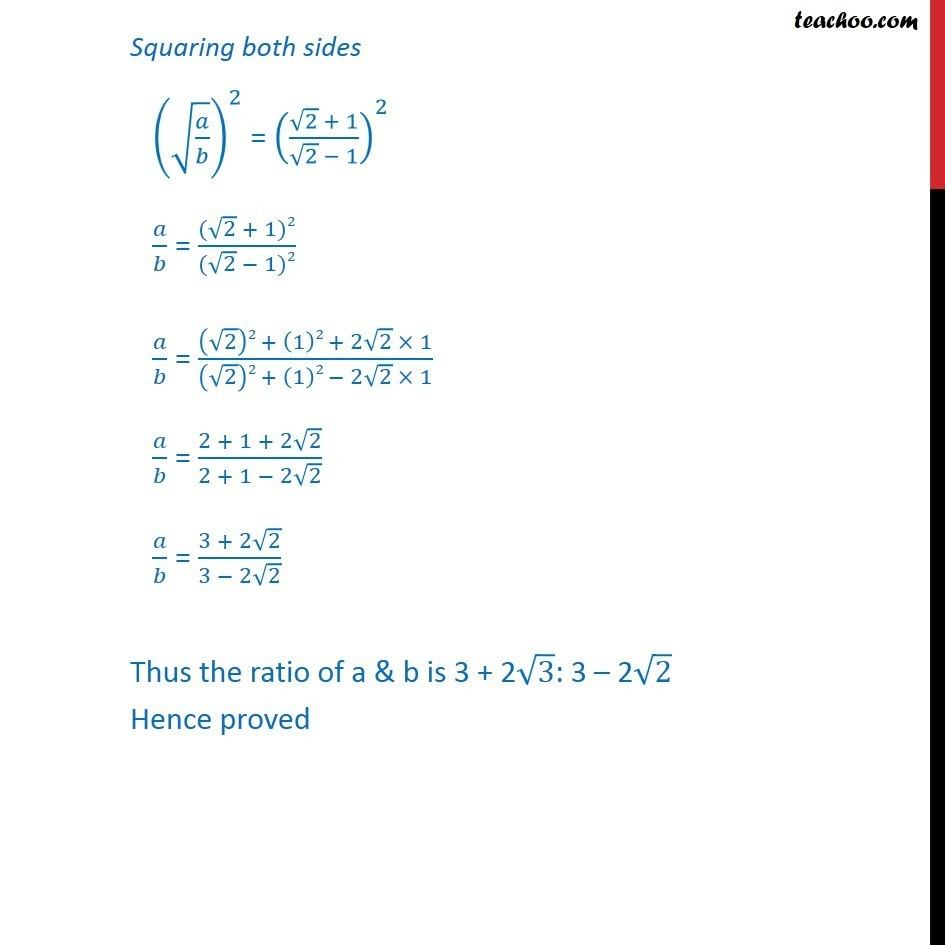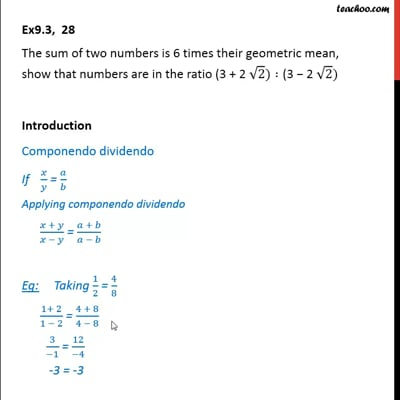This video is only available for Teachoo black users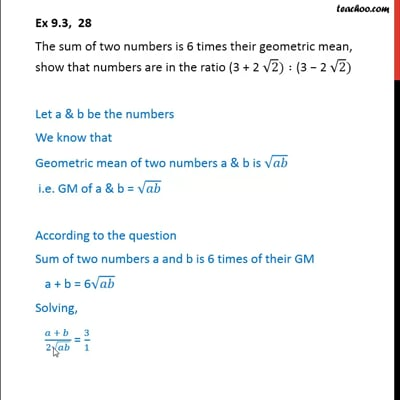This video is only available for Teachoo black users

### Transcript

Ex9.3, 28 The sum of two numbers is 6 times their geometric mean, show that numbers are in the ratio (3 + 2 2) :"(3 2 " 2) Introduction Componendo dividendo If / = / Applying componendo dividendo ( + )/( ) = ( + )/( ) Eg: Taking 1/2 = 4/8 (1+ 2)/(1 2) = (4 + 8)/(4 8) 3/( 1) = 12/( 4) -3 = -3 Ex 9.3, 28 The sum of two numbers is 6 times their geometric mean, show that numbers are in the ratio (3 + 2 2) :"(3 2 " 2) Let a & b be the numbers We know that Geometric mean of two numbers a & b is i.e. GM of a & b = According to the question Sum of two numbers a and b is 6 times of their GM a + b = 6 Solving, ( + )/(2 ) = 3/1 Applying componendo & dividendo ( + +2 )/( + 2 ) = (3 + 1)/(3 1 ) (( )2+( )2+2( ))/(( )2+( )2 2( ) ) = 4/2 Using (x + y)2 = x2 + y2 + 2xy (x - y)2 = x2 + y2 - 2xy ( + )2/( )2 = 2/1 (( + )/( ))^2 = 2/1 ( + )/( ) = 2/( 1) Again applying componendo & dividendo (( + )+( ))/(( + ) ( ) ) = ( 2 + 1)/( 2 1) ( + + )/( + + ) = ( 2 + 1)/( 2 1) (2 + 0)/( + + ) = ( 2 + 1)/( 2 1) (2 )/(2 + 0) = ( 2 + 1)/( 2 1) (2 )/(2 ) = ( 2 + 1)/( 2 1) ( / ) = ( 2 + 1)/( 2 1) Squaring both sides ( ( / ))^2 = (( 2 + 1)/( 2 1))^2 / = (( 2 + 1)2)/(( 2 1)2) / = (( 2)2 + (1)2 + 2 2 1)/(( 2)2 + (1)2 2 2 1) / = (2 + 1 + 2 2)/(2 + 1 2 2) / = (3 + 2 2)/(3 2 2) Thus the ratio of a & b is 3 + 2 3: 3 2 2 Hence proved